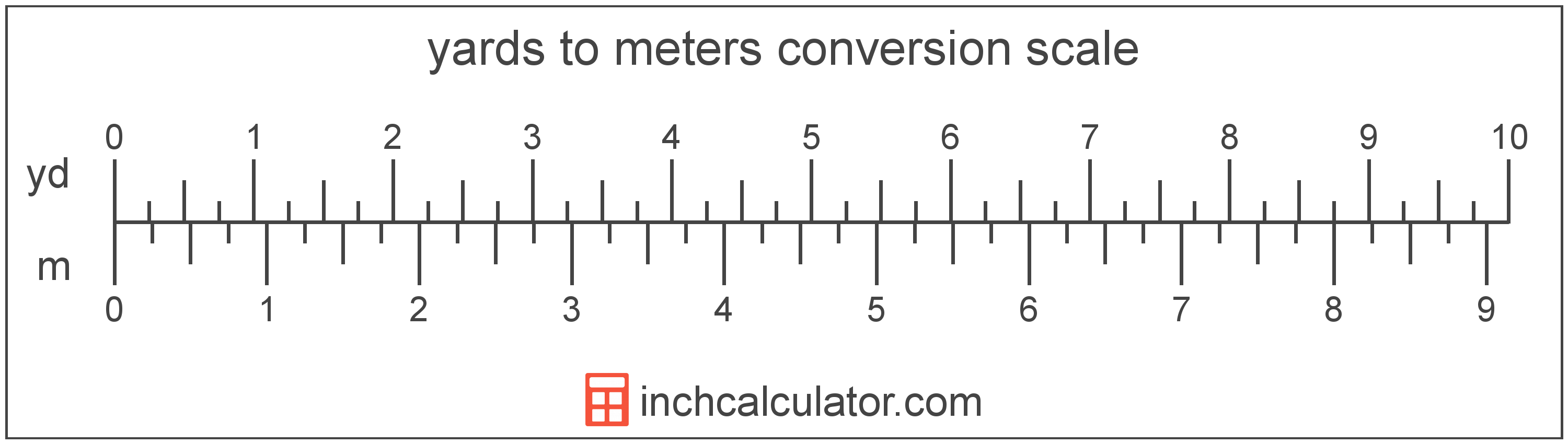# Yards to Meters Converter

Enter the length in yards below to get the value converted to meters.

yd
ft
Results in Meters:1 yd = 0.9144 m

Do you want to convert meters to yards?

## How to Convert Yards to Meters

To convert a measurement in yards to a measurement in meters, multiply the length by the following conversion ratio: 0.9144 meters/yard.

Since one yard is equal to 0.9144 meters, you can use this simple formula to convert:

meters = yards × 0.9144

The length in meters is equal to the length in yards multiplied by 0.9144.

For example, here's how to convert 5 yards to meters using the formula above.
meters = (5 yd × 0.9144) = 4.572 m### How Many Meters Are in a Yard?

There are 0.9144 meters in a yard, which is why we use this value in the formula above.

1 yd = 0.9144 m

## What is a Yard?

The yard is a unit of length measurement equal to 3 feet or 36 inches. The international yard is legally defined to be equal to exactly 0.9144 meters.

The yard is a US customary and imperial unit of length. Yards can be abbreviated as yd; for example, 1 yard can be written as 1 yd.

## What is a Meter?

According to the most recent 2019 definition, the meter is defined as the distance traveled by light in vacuum during a time interval with a duration of 1/299,792,458 of a second. One meter is equal to 100 centimeters, 3.28084 feet, or 39.37 inches.

The meter, or metre, is the SI base unit for length in the metric system. Meters can be abbreviated as m; for example, 1 meter can be written as 1 m.

We recommend using a ruler or tape measure for measuring length, which can be found at a local retailer or home center. Rulers are available in imperial, metric, or a combination of both values, so make sure you get the correct type for your needs.

Need a ruler? Try our free downloadable and printable rulers, which include both imperial and metric measurements.

## Yard to Meter Conversion Table

Table showing various yard measurements converted to meters.
Yards Meters
1 yd 0.9144 m
2 yd 1.8288 m
3 yd 2.7432 m
4 yd 3.6576 m
5 yd 4.572 m
6 yd 5.4864 m
7 yd 6.4008 m
8 yd 7.3152 m
9 yd 8.2296 m
10 yd 9.144 m
11 yd 10.06 m
12 yd 10.97 m
13 yd 11.89 m
14 yd 12.8 m
15 yd 13.72 m
16 yd 14.63 m
17 yd 15.54 m
18 yd 16.46 m
19 yd 17.37 m
20 yd 18.29 m
21 yd 19.2 m
22 yd 20.12 m
23 yd 21.03 m
24 yd 21.95 m
25 yd 22.86 m
26 yd 23.77 m
27 yd 24.69 m
28 yd 25.6 m
29 yd 26.52 m
30 yd 27.43 m
31 yd 28.35 m
32 yd 29.26 m
33 yd 30.18 m
34 yd 31.09 m
35 yd 32 m
36 yd 32.92 m
37 yd 33.83 m
38 yd 34.75 m
39 yd 35.66 m
40 yd 36.58 m

## References

1. National Bureau of Standards, Refinement of Values for the Yard and the Pound, https://www.ngs.noaa.gov/PUBS_LIB/FedRegister/FRdoc59-5442.pdf
2. International Bureau of Weights and Measures, The International System of Units, 9th Edition, 2019, https://www.bipm.org/documents/20126/41483022/SI-Brochure-9-EN.pdf